Before you start, have you read the introduction to the Ternary package?

This guide gives a brief example of using the “Ternary” package, using road casualty data from Great Britain.

First, we’ll load and preview the data:

``````data("Seatbelts")

# View the first few entries:
``````##      DriversKilled drivers front rear   kms PetrolPrice VanKilled law
## [1,]           107    1687   867  269  9059   0.1029718        12   0
## [2,]            97    1508   825  265  7685   0.1023630         6   0
## [3,]           102    1507   806  319  9963   0.1020625        12   0
## [4,]            87    1385   814  407 10955   0.1008733         8   0
## [5,]           119    1632   991  454 11823   0.1010197        10   0
## [6,]           106    1511   945  427 12391   0.1005812        13   0``````

For our example study, we’ll explore the relative safety of car passengers through time by plotting the proportion of people killed or seriously injured in accidents who were drivers (column `drivers`), front-seat passengers (column `front`), or rear-seat passengers (column `drivers`).

``````# Load the Ternary library
library("Ternary")

# Define our columns of interest:
seat <- c("drivers", "front", "rear")

# Extract and plot the data
TernaryPlot(alab = seat, blab = seat, clab = seat)
TernaryPoints(Seatbelts[, seat])``````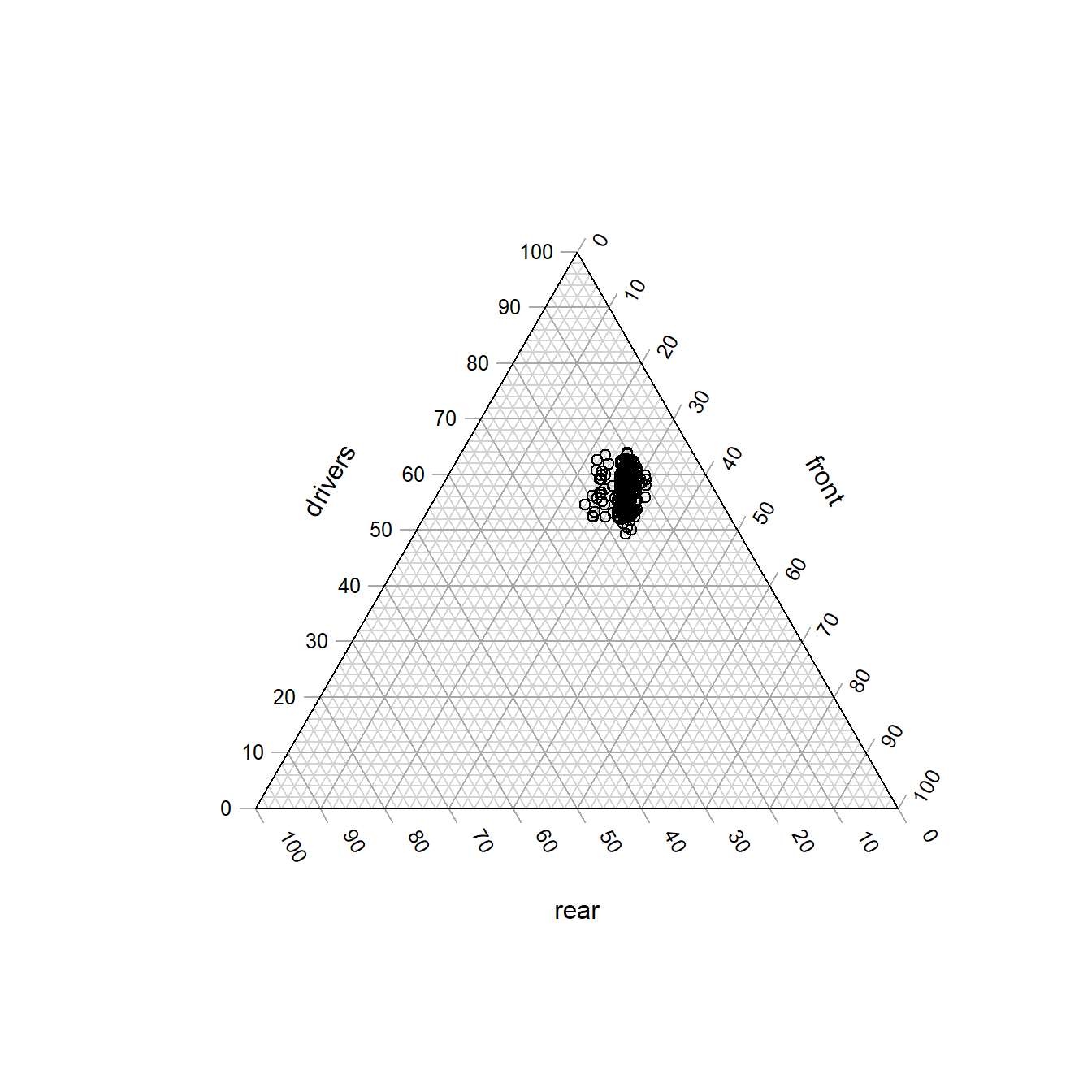The `law` column takes the value `1` for months in which the wearing of a seatbelt was made compulsory in UK law. Let’s explore the impact of this law by plotting data before and after the law with different symbols. R’s plotting characters are numbered thus:

``````par(mar = c(0, 0, 0, 0))
plot(0:20, rep(2, 21), pch = 0:20,
cex = 2, ylim = c(-1, 3), ann = FALSE)
text(0:20, rep(0, 21), 0:20)``````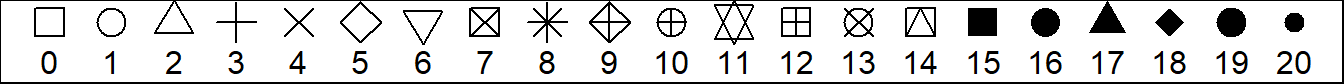Let’s choose to plot a `+` (character `3`) when seatbelts are legally required, and `o` (character `1`) when they are not.

``beltLawPch <- ifelse(Seatbelts[, "law"], 3, 1)``

Let’s also make the plot more readable by shrinking the margins:

``````# Shrink the margin so the plot's easier to read
par(mar = c(0, 0, 0, 0))

# Set up a blank plot
TernaryPlot(alab = seat, blab = seat, clab = seat)

legend("topleft", c("Belt law", "No law"), pch = c(3, 1))

# Use our beltLawPch variable to style points
TernaryPoints(Seatbelts[, seat], pch = beltLawPch)``````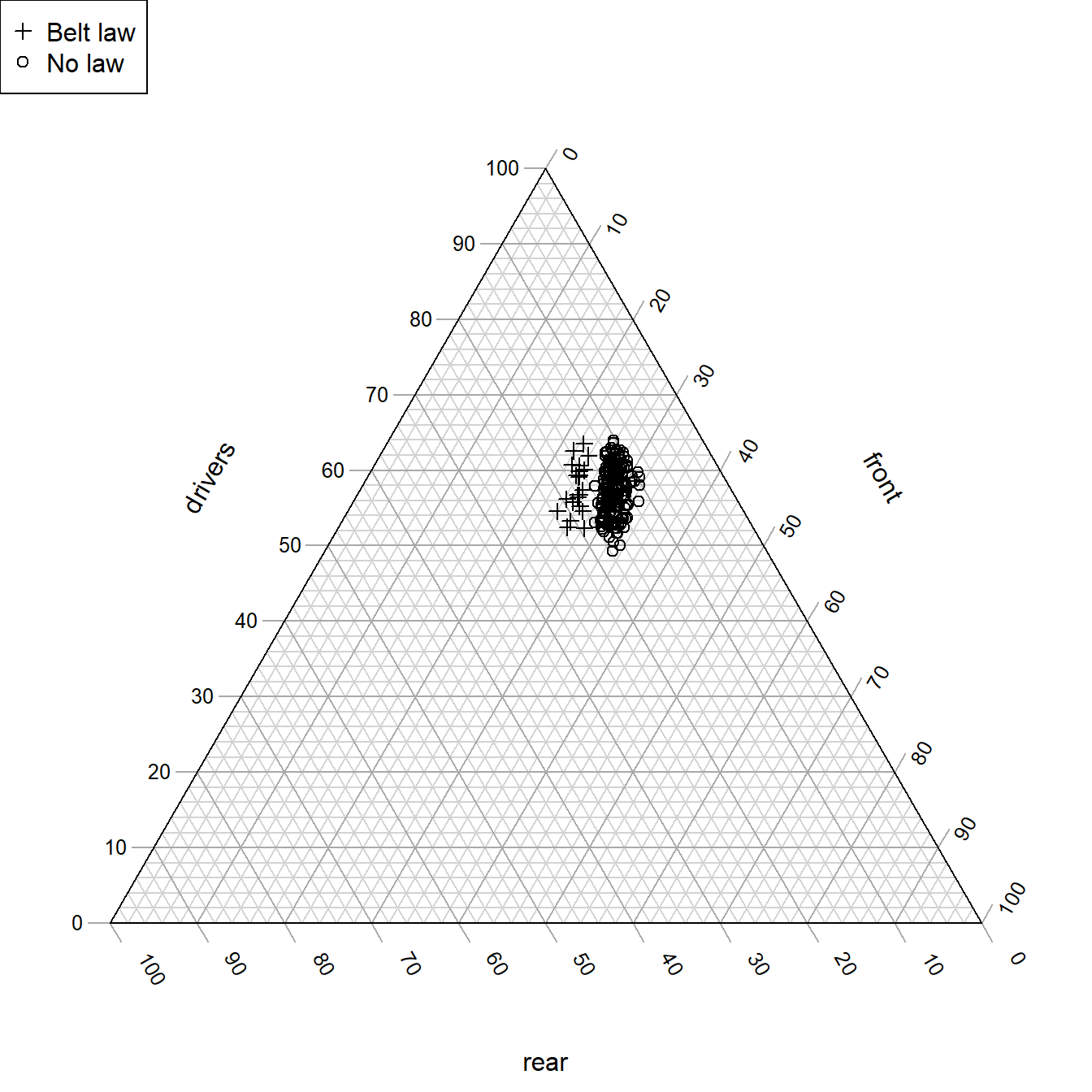This seems to show a clear pattern: the seatbelt law led to a higher proportion of deaths and serious injuries occurring in the rear of cars.

Let’s look for other trends through time by colouring each data point by when it was collected. Each row summarizes data for a consecutive month, so we can simply colour the points by row number.

Let’s define a spectrum. The “viridis” colour scale is free from perceptual artefacts, is colour-blind friendly, and works when printed in greyscale.

``````nPoints <- nrow(Seatbelts)
rowCol <- hcl.colors(nPoints, palette = "viridis", alpha = 0.8)``````

Now we can plot our data:

``````par(mar = c(0, 0, 0, 0))

TernaryPlot(alab = seat, blab = seat, clab = seat)
legend("topleft", c("Belt law", "No law"), pch = c(3, 1))

# Add a legend for our colour scale
PlotTools::SpectrumLegend(palette = rowCol, legend = c("Dec 1984", "Jan 1969"))

# Use our rowCol variable to style points
TernaryPoints(Seatbelts[, seat], pch = beltLawPch,
lwd = 2, # Use wider lines so points are clearer
col = rowCol)``````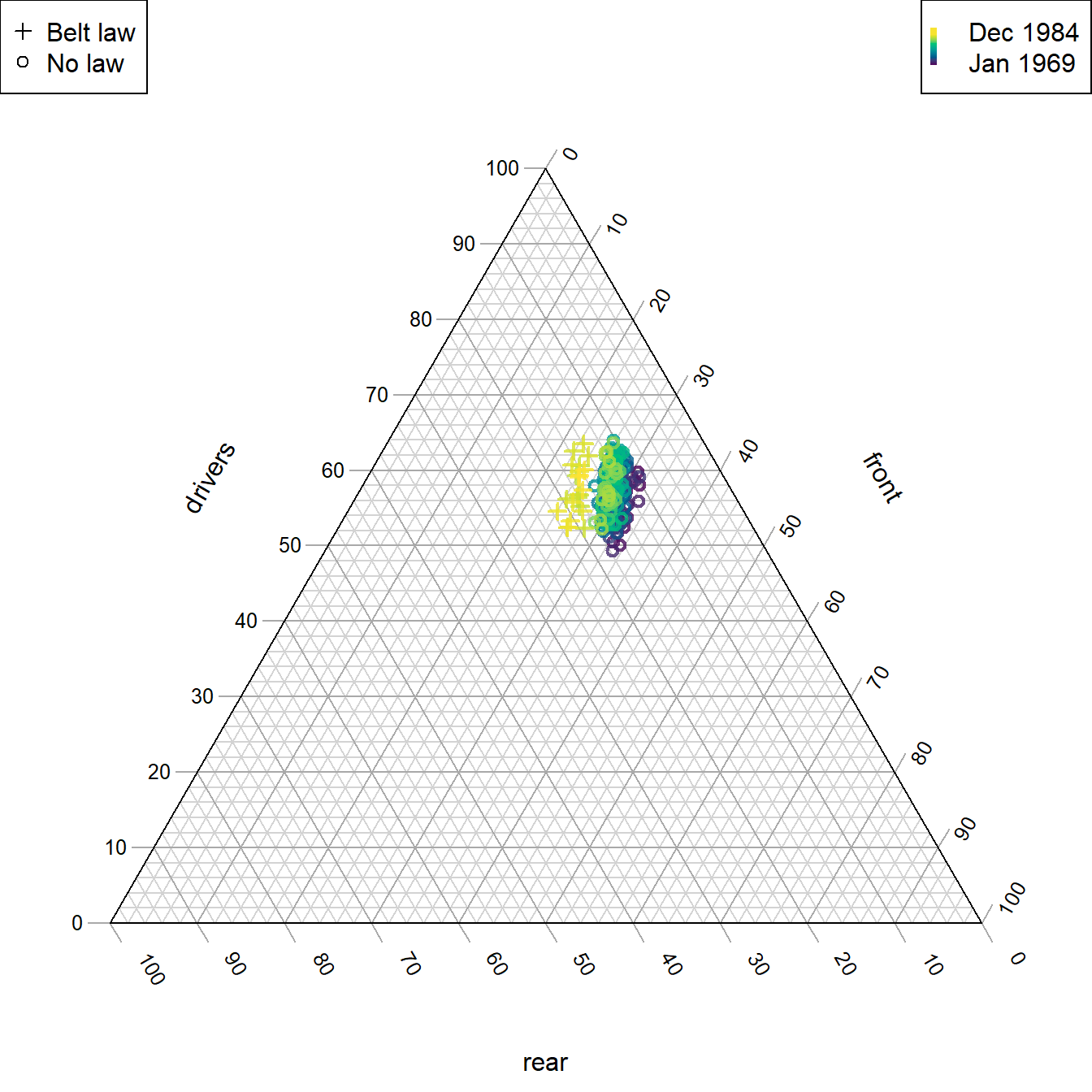Our belt law appears to sit in the context of a longer term improvement in the relative safety of front-seat passengers. But how do we explain the scatter in the ‘drivers’ dimension? Let’s try plotting seasonal variation:

``````# Define a suitable cyclical spectrum
fourSeasons <- hcl.colors(4, "Spectral")
monthCol <- colorRampPalette(fourSeasons[c(1:4, 1)])(13)[c(7:12, 1:6)]

par(mar = c(0, 0, 0, 0))

TernaryPlot(alab = seat, blab = seat, clab = seat)
legend("topleft", c("Belt law", "No law"), pch = c(3, 1))

legendMonths <- seq(1, 12, by = 2)
# Add a legend for our colour index
legend(
"topright",
bty = "n", # No frame
pch = 15, # Filled square
col = monthCol,
legend = month.abb,
title = "Month"
)

# Style points by law and month
TernaryPoints(Seatbelts[, seat], pch = beltLawPch,
lwd = 2, # Use wider lines so points are clearer
col = monthCol)``````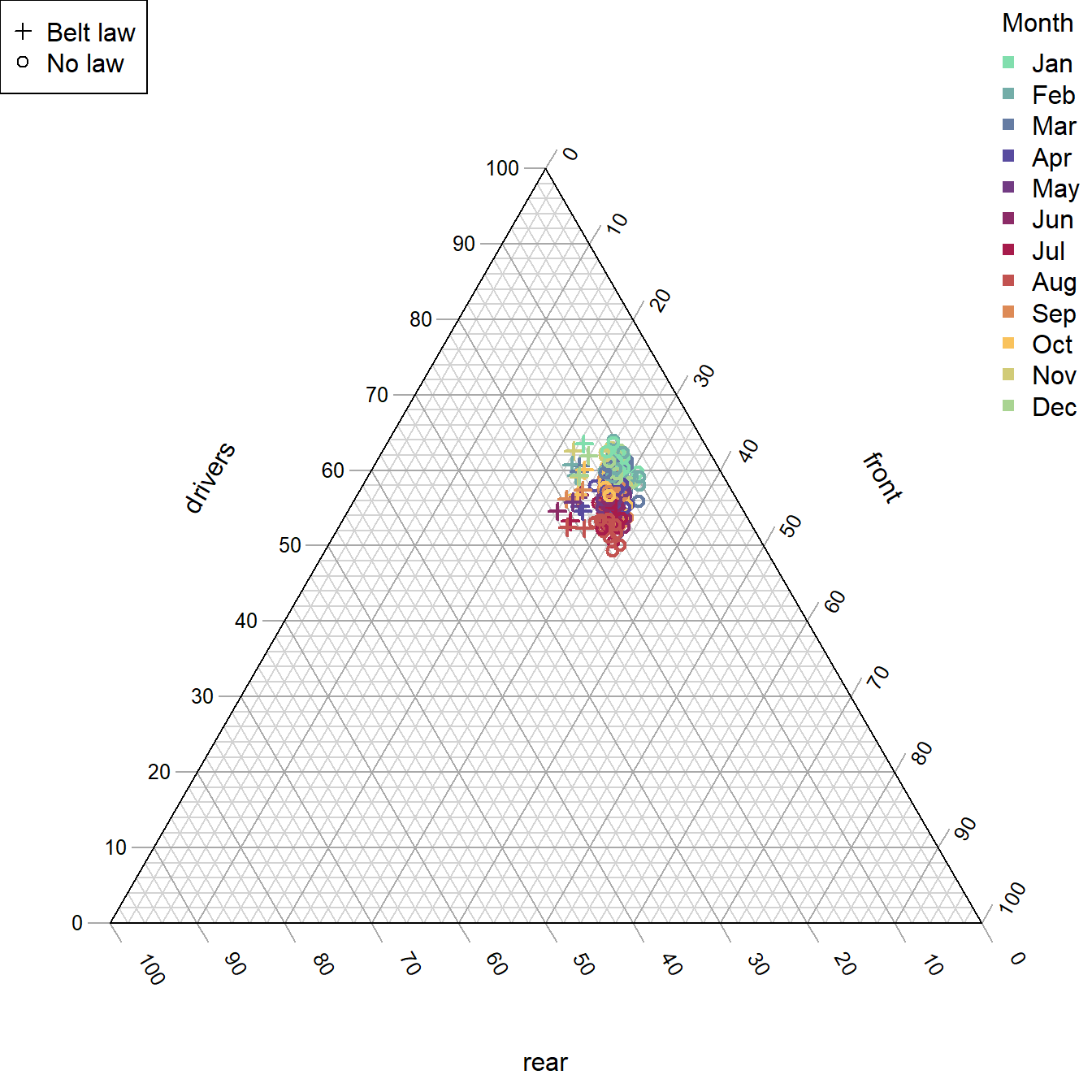With or without seatbelts, drivers are in more relative danger in winter!

Now let’s size each point by the number of casualties, scaled by distance driven. We’ll need to zoom in closer to the plot to see this clearly.

``````par(mar = c(0, 0, 0, 0))

TernaryPlot(alab = seat, blab = seat, clab = seat,
# Magnify the "action":
xlim = c(0.055, 0.095), ylim = c(0.48, 0.52))

legend("topleft", c("No law", "Belt law"),
col = 2:3, pch = 1, lwd = 2, lty = NA)
sizes <- c(3, 7, 12)
scale <- 200
legend("topright", title = "Casualties / Mm", legend = sizes,
pt.cex = sizes / 1000 * scale,
pch = 1, lwd = 2, lty = NA)

# Style points according to casualties and the law
TernaryPoints(Seatbelts[, seat], pch = 1, lwd = 2,
cex = Seatbelts[, "DriversKilled"] / Seatbelts[, "kms"] * scale,
col = 2 + Seatbelts[, "law"])``````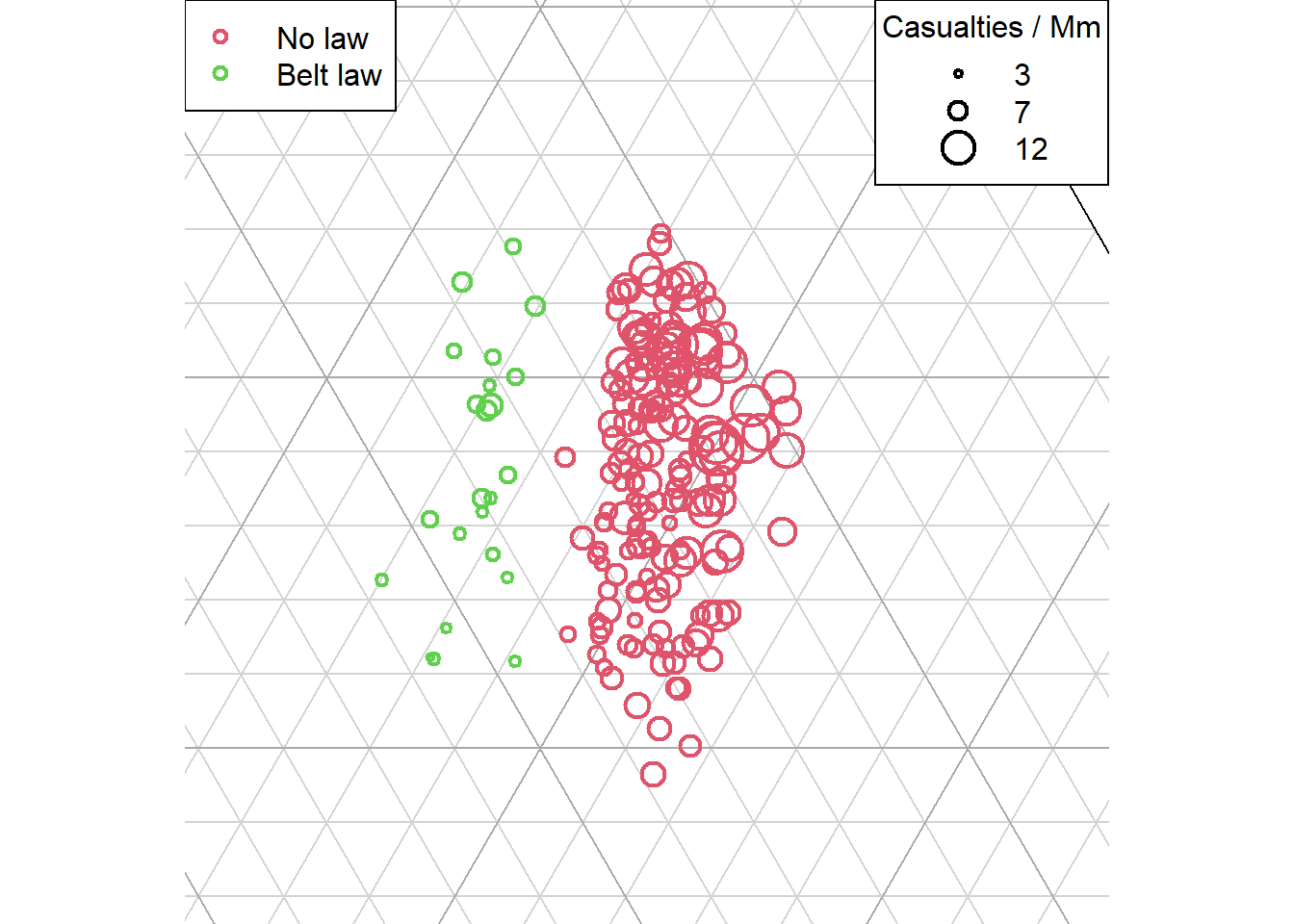The smaller size of points after mandatory seatbelt usage is evident.

Now let’s plot the annual trend as a line, taking the values for each October as representative:

``````# Subset our data to extract only Octobers:
oct <- month.name == "October"
octBelts <- Seatbelts[oct, ]

par(mar = c(0, 0, 0, 0))
TernaryPlot(alab = seat, blab = seat, clab = seat,
xlim = c(0.055, 0.095), ylim = c(0.48, 0.52),

TernarySegments(octBelts[-nrow(octBelts), seat], octBelts[-1, seat],
col = rowCol, lwd = 2)

# Label each point by its year
TernaryText(octBelts[, seat], paste0("'", 69:84),
font = 2, cex = 1.5,
# Semi-transparent colours
col = adjustcolor(rowCol[oct], alpha.f = 0.8))

# Provide a legend for our colour scheme
PlotTools::SpectrumLegend(
"topright",
bty = "n",
palette = rowCol,
legend = seq(1984, 1969, length.out = 4),
title = "Year"
)``````